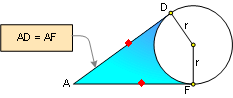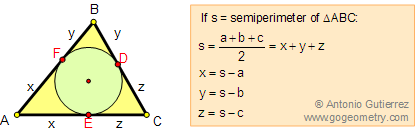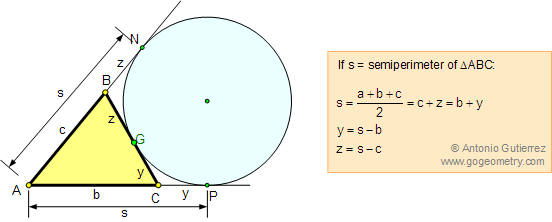# Geometry Problem 86. Intouch and Extouch Triangles, Equal Area, Contact Triangles. High School, College, Math Education

 In the figure below, given a triangle ABC, Si is the area of the contact or intouch triangle DEF, Se is the area of the extouch triangle GHM. Prove that:.HINTS: 1. The contact triangle of a triangle ABC, also called the intouch triangle or Gergonne triangle, is the triangle DEF formed by the points of tangency of the incircle of triangle ABC with triangle ABC. 2. The extouch triangle of a triangle ABC is the triangle GHM formed by the points of tangency of the triangle ABC with its excircles.   3. TANGENT TO A CIRCLE Proposition. Two tangent segments to a circle from an external point are congruent.4. Semiperimeter s, Side and Incircle Formula5. Semiperimeter s, Side and Excircle Formula6. AREA OF A TRIANGLE: Proposition: The area of a triangle equals one-half the product of the length of a side and the length of the altitude to that side. Side Angle Side Formula: The SAS formula = ½ (side1 × side2) × sine(included angle).6. See Problems 82, 85.
 Home | Search | Geometry | Problems | All Problems | Open Problems | Visual Index | Art | 81-90 | Circle Tangent Line | Email | View or post a solution | by Antonio Gutierrez